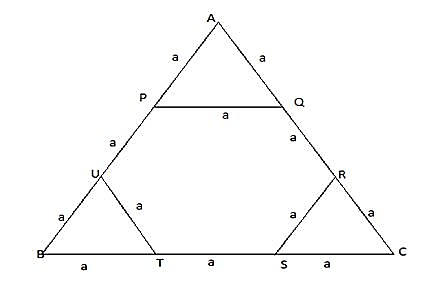### CAT 2019 – Slot 1 – Quantitative Ability – If m and n are integers such that

Q. 1: If m and n are integers such that (√2)^19 * 3^4 * 4^2 * 9^m * 8^n = 3^n * 16^m (4√64) then m is?

1. -20
2. -24
3. -16
4. -12

Given, √2^19 3^4 4^2 9^m 8^n=3^n 16^m ∜64

Or 2^(19/2)×3^4×2^4×3^2m×2^3n= 3^n×2^4m×2^(6/4)

2^(19/2+4+3n)×3^(4+2m)=3^n×2^((4m+6/4) )

Comparing both sides,

n = 4+2m

19/2 +4 + 3n = 4m + 6/4

Or (19 + 8)/2 + 3(4+2m) = 4m + 3/2

27/2 + 12 + 6m = 4m + 3/2

6m -4m = 3/2 – 27/2 -12

2m = -24

Thus m = -12

##### 1. 282. 313. 294. 32

Let the income of Amala (A) = 100x , income of Bimala (B) = 100x/1.2 = 83.33 x and
Income of Kamala = 100x/0.8 = 125x
When Kamala’s income goes down by 4% and Bimala’s goes up by 10%,
K’s new income = 125x – 4% of 125x = 120x
B’s new income = 83.33x + 10% of 83.33x = 91.67 x
the percentage by which Kamala’s income would exceed Bimala’s = (120x – 91.67x)/91.67x *100
= (28.33/91.67) *100 = 31 (approx)

Q. 3: In a class, 60% of the students are girls and the rest are boys. There are 30 more girls than boys. If 68% of the students, including 30 boys, pass an examination, the percentage of the girls who do not pass is

let total number of students in the class = 100x
Number of girls = 60% of 100x = 60x
Number of boys = 100x – 60x = 40x
Given, 60x – 40x = 30
So 20x = 30
x = 30/20
thus number of students = 100x = 100*30/20 = 150
number of boys = 40x = 40*30/20 = 60
number of girls = 150 – 60 =90
number of students who do not pass the exams = 32% of 100x = 32x = 32*30/20 = 48
number of boys failed= 60 -30 = 30
so number of girls failed = 48 – 30 = 18
required percentage = 18/90 *100 = 20%

Q. 4: On selling a pen at 5% loss and a book at 15% gain, Karim gains Rs. 7. If he sells the pen at 5% gain and the book at 10% gain, he gains Rs. 13. What is the cost price of the book in Rupees?
1. 95
2. 100
3. 80
4. 85

let the CP of pen = p and that of book = b
So as per question 0.15 b – 0.05 p = 7
Or 15b – 5p = 700 ————-1)
Similarly 10b + 5p = 1300 —–2)
Adding both equations 25b = 2000
Or b = 2000/25 = 80

Q. 5: Corners are cut off from an equilateral triangle T to produce a regular hexagon H. Then, the ratio of the area of H to the area of T is

1. 3 : 4
2. 2 : 3
3. 5 : 6
4. 4 : 5

In given case , figure can be drawn as belowLet side of equilateral triangle ABC = 3a

So side of hexagon = a

Area of triangle = (root3 /4 )*(3a)^2 = 9a^2 *(root3/4)

Area of hexagon with side a = 6*(root3 /4 )*(a)^2

Ratio of areas of hexagon to that of triangle = { 6*(root3 /4 )*(a)^2 } / { 9a^2 *(root3/4)} = 6/9 = 2/3

Thus required ratio = 2 : 3

Inspiring Education… Assuring Success!!

Ghatkopar | Borivali | Andheri | Online